# Circle + sphere - math problems

#### Number of problems found: 15

• Sphere cutsAt what distance from the center intersects sphere with radius R = 56 plane, if the cut area and area of the main sphere circle is in ratio 1/2.
• Sphere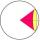Intersect between plane and a sphere is a circle with a radius of 60 mm. Cone whose base is this circle and whose apex is at the center of the sphere has a height of 34 mm. Calculate the surface area and volume of a sphere.
• Inscribed circle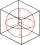A circle is inscribed at the bottom wall of the cube with an edge (a = 1). What is the radius of the spherical surface that contains this circle and one of the vertex of the top cube base?
• Sphere in coneA sphere is inscribed in the cone (the intersection of their boundaries consists of a circle and one point). The ratio of the surface of the ball and the contents of the base is 4: 3. A plane passing through the axis of a cone cuts the cone in an isoscele
• Sphere parts, segmentA sphere with a diameter of 20.6 cm, the cut is a circle with a diameter of 16.2 cm. .What are the volume of the segment and the surface of the segment?
• Horizon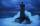The top of a lighthouse is 19 m above the sea. How far away is an object which is just “on the horizon”? [Assume the earth is a sphere of radius 6378.1 km.]
• The EarthThe Earth's surface is 510,000,000 km2. Calculates the radius, equator length, and volume of the Earth, assuming the Earth has the shape of a sphere.
• Elevation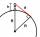What must be the elevation of an observer in order that he may be able to see an object on the earth 536 km away? Assume the earth to be a smooth sphere with radius 6378.1 km.
• Earth's circumference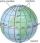Calculate the Earth's circumference of the parallel 48 degrees and 10 minutes.
• Earth parallelEarth's radius is 6370 km long. Calculate the length parallel of latitude 50°.
• Average speed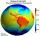What is the average speed you have to move the way around the world in 80 days? (Path along the equator, round to km/h).
• MO SK/CZ Z9–I–3John had the ball that rolled into the pool, and it swam in the water. Its highest point was 2 cm above the surface. The diameter of the circle that marked the water level on the surface of the ball was 8 cm. Find the diameter of John ball.
• MoonWe see Moon in the perspective angle 28'. Moon's radius is 1740 km at the time of the full moon. Calculate the mean distance of the Moon from the Earth.
• What is bigger?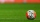Which ball has a larger volume: a football with a circumference of 66 cm or a volleyball with a diameter of 20 cm?
• Float boya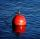A 0.5 meter spherical float is used as a location mark for a fishing boat anchor. It floats in salt water. Find the depth to which the float sinks if the material of which the float is made weighs 8 kilograms per cubic meter and salt water weighs 1027 kg/

Do you have an interesting mathematical word problem that you can't solve it? Submit a math problem, and we can try to solve it.

We will send a solution to your e-mail address. Solved examples are also published here. Please enter the e-mail correctly and check whether you don't have a full mailbox.

Please do not submit problems from current active competitions such as Mathematical Olympiad, correspondence seminars etc...

Circle Problems. Sphere Problems.